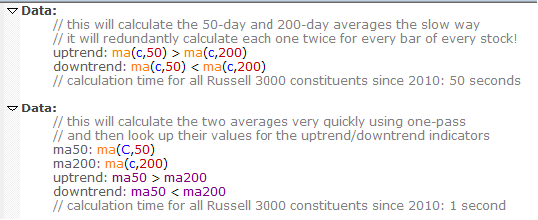Navigation: Realtest Script Language > Script Sections > Data Section > One-Pass Data Formulas

The following material is kept here for reference and/or curiosity, but it is no longer necessary to understand and follow these guidelines.

Functions and indicators that can support one-pass calculation are now automatically calculated this way even when they're embedded within a larger formula expression.

A regular Data Section item formula is calculated by looping through all the dates of the symbol and evaluating the formula for each date. If the formula includes an indicator or rolling bar function with a long lookback length, this can take some time to accomplish, since it has to "roll back" that many bars from each bar of each symbol.

For most types of formulas, this can't be avoided, and fortunately RealTest already performs these calculations as quickly as possible, using multiple threads.

For certain commonly-used functions and indicators, it is possible to dramatically speed up their calculation if they are structured and declared a certain way.

The difference in speed between the standard calculation method and "one-pass" is roughly a factor of one third of the lookback length. For example, a 100-day moving average can be calculated approximately 33 times faster when the one-pass optimization can be applied.

When you think about a backtest universe with 10,000 stocks going back 20 years (5000 daily bars per stock), you can start to imagine how much faster long-lookback indicators can be calculated if you structure your scripts to take advantage of one-pass mode.

All of the functions and indicators listed below will be automatically calculated in one pass if and only if the following conditions are all true:

the indicator is the only element of the data item formula (not part of a larger expression)

the length argument is a constant (literal number) or a parameter reference

For example, below are two ways to calculate simple uptrend / downtrend variables:In the first Data section above, RealTest cannot use one-pass calculation for either formula, because they each contain two functions and an operator.

In the second Data section, the moving averages are each given their own data items, which allows them to be calculated using one-pass.

The following functions and indicators support one-pass calculation if they are specified as described above:

ATR

MDI

PDI

RSI

RSIF

RRSI

BBTOP

BBBOT

KBBOT

KBTOP

MACD

MACDS

MACDH

SUM

MA / AVG

EMA / XAVG

Highest / HHV

Lowest / LLV

SinceHigh

SinceLow

StdDev

Skewness

Kurtosis

HVOL

Additionally, the following functions support one-pass calculation when their optional length or "nth" argments are NOT present:

CountTrue

TrueInRow

SinceTrue

UntilTrue

WhenTrue

SumSince

It is worth your while to carefully structure your Data Section so that long-lookback indicators can take advantage of one-pass calculation!

To do this most effectively, look through all of your data and strategy formulas to find any of the above indicators, give each unique one its own data item, and change all the places where it is used to references to that data item.

The bensdorp_book.rts example script gives an excellent illustration of this technique. Imagine (or test, if you're curious) how much more slowly that set of seven strategies would run without the one-pass calculations!